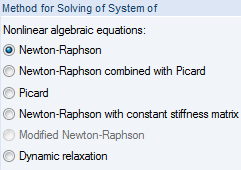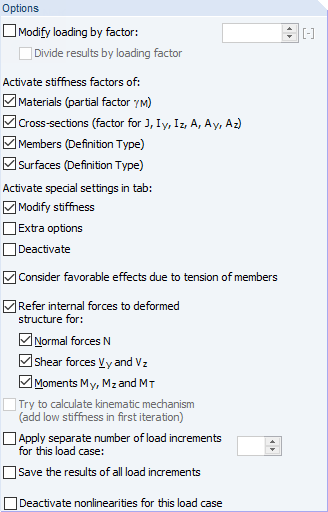# RFEM – Online Manual

Online manuals, introductory examples, tutorials, and other documentation.

# 7.3.1.1 Calculation Parameters

#### Calculation Parameters

Method of Analysis

This dialog section allows you to control whether the load case/combination is calculated according to the Geometrically linear static, Second-order, or Large deformation analysis. Select the Postcritical analysis option to carry out a stability analysis according to the large deformation analysis with regard to postcritical failure of the entire structure.

RFEM presets the linear calculation according to the linear static analysis for load cases and the nonlinear calculation according to the second-order analysis for load combinations.

If the model includes cable members, the calculation according to the large deformation analysis is preset in all cases.

Second-order analysis

The common "structural" second-order analysis is used to determine the equilibrium on the deformed system. Deformations are assumed to be small. If axial forces are available in the system, they lead to an increase in bending moments. Thus, the calculation according to the second-order analysis by Timoshenko  only has an effect if axial forces are considerably higher than shear forces. The additional bending moment ΔM results from the axial force N and the elastic lever eel.

For structural systems subjected to pressure, there is an overlinear relation between loading and internal forces. Normally, you also have to calculate with γ-fold actions.

The axial force difference in the iterations represents the break-off criterion. For member elements, the stiffness-modifying axial force decisive for the second-order analysis is assumed to be constant along the entire member. The calculation stops as soon as you fall short of a certain value of the normal force difference. It is possible to influence this break-off limit in the Precision and Tolerance dialog section of the Global Calculation Parameters dialog tab.

For nonlinear calculations according to the second-order analysis, assumptions of the linear elastic analysis are the same, with the following additions:

• No plastic deformations occur.
• The external forces stay true to the direction.
• For members with a non-constant axial force (e.g. columns), the mean value of the axial force N is applied for determining the member coefficient ε.
Large deformation analysis

The large deformation analysis ("third-order theory") takes longitudinal and transversal forces into account during the analysis of internal forces. If the calculation according to the large deformation analysis is selected, all surfaces and members are calculated according to this calculation theory.

After each iteration step, the stiffness matrix for the deformed system is created. There are significant differences between loads that are defined as local and global: For example, if a surface load defined as global in Z acts on a floor, it keeps its direction if the finite elements warp. But when the load is effective in direction of the local surface axis z, it warps on each element according to the element's warping.

Postcritical analysis

A stability analysis with regard to postcritical failure is performed. The method represents a modified calculation according to the large deformation analysis by Newton-Raphson where the influence of axial forces is considered for changes in shear and bending stiffness. The tangential stiffness matrix is saved in each iteration step. If there are singularities (i.e. an instability), the stiffness matrix of the previous iteration step is used for new, geometric, incremental iterations until the tangential stiffness matrix of the current setting becomes regular (stable).

Method for solving of system of nonlinear equationsThere are six available methods for solving the nonlinear, algebraic system of equations:

Newton-Raphson

The approach according to Newton-Raphson is preset for the large deformation analysis. The nonlinear equation system is solved numerically by means of iterative approximations with tangents. The tangential stiffness matrix is determined as a function of the current state of deformation; it is inverted in each cycle of iterations. In the majority of cases, a fast (quadratic) convergence is reached.

You can influence the convergence behavior with the number of load increments set in the Global Calculation Parameters dialog tab.

Newton-Raphson combined with Picard

First, the approach according to Picard is applied (see below). After a few iterations, the program changes to the Newton-Raphson method. The basic idea of this approach is to use the relatively strong Picard method for the first iteration steps to avoid instability messages. This initial approximation is followed by the fast method according to Newton-Raphson to find the ultimate state of equilibrium.

In the Settings dialog section of the Global Calculation Parameters dialog tab, you can define the percentage that is used for iterations according to Picard when the combined method is applied (see Figure 7.25).

Picard

The method according to Picard, also known as secant method, can be understood as a finite difference approximation of the Newton method. The program takes the difference between the current and original iteration run in the current load increment step into account.

Often, converging is slower than in the calculation method according to Newton-Raphson. But the method proves to be stronger in regard to nonlinear problems, making the calculation more stable.

Newton-Raphson with constant stiffness matrix

This version of the Newton-Raphson method can be selected for calculations according to the large deformation analysis. The stiffness matrix is created only once in the first iteration step and then used in all subsequent calculation loops.

Thus, the calculation runs faster but is not as stable as calculations according to the normal or modified Newton-Raphson method.

Modified Newton-Raphson

This method is used to perform the postcritical analysis (see Method of Analysis dialog section above) where a range with instability must be overcome. If an instability is available and the stiffness matrix cannot be inverted, the program uses the stiffness matrix of the last stable iteration step. The program continues to calculate with this matrix until a stability range is reached again.

Dynamic relaxation

The final method is suitable for calculations according to the large deformation analysis and for solving problems regarding the postcritical analysis. In this approach, an artificial time parameter is introduced. Taking inertia and damping into account, the failure can be handled as a dynamic problem. This approach uses the explicit time-integration method; the stiffness matrix is not inverted. No part of the model is allowed to have a specific weight of zero when calculating with dynamic relaxation.

This method includes the Rayleigh damping that can be defined by means of the constants α and β according to the following equation with derivations by time:

where

• M : Mass matrix

• C : Damping matrix C = α M + β diag(K)

• K: Stiffness matrix

• f : Vector of external forces

• u : Discretized displacement vector
OptionsModify loading by factor

After selecting the check box, you can enter a factor into the text box, which all the loads contained in the load case or combination will be multiplied with (does not apply to imperfections). The factor is also reflected in the load vectors and values of the graphic. Generally, negative factors are also permitted.

Older standards claim to multiply loads globally by a certain factor in order to increase effects for stability designs according to the second-order analysis. On the other hand, design must be carried out with the characteristic loads. Both requirements can be fulfilled by entering a factor larger than 1.00 and selecting the Divide results by loading factor check box.

When analyzing structures according to current standards, loading should not be edited with any factors. Instead, partial safety factors and combination coefficients must be applied for the superposition in the load and result combinations.

Activate stiffness factors

With these check boxes, you can consider the stiffness factors of the materials (see Chapter 4.3), cross-sections (see Chapter 4.13), members (see Chapter 4.17), and surfaces (see Chapter 4.4) in the calculation.

Activate special settings

When selecting the Modify stiffnesses, Extra options, and Deactivate check boxes, additional tabs are accessible. You can set specific requirements for stiffnesses (see Chapter 7.3.1.2) and Objects (see Chapter 7.3.1.4) or activate the initial deformations of a load case or the results of an add-on module for the calculation (see Chapter 7.3.1.3).

Consider favorable effects due to tension of members

Tensile forces have a favorable effect on pre-deformed structural systems. Thus, the pre-deformation is reduced and the structure is stabilized.

There are different opinions on how to consider tensile forces that act in a favorable way. The standards contain regulations, which stipulate that relieving actions must be considered with a smaller partial safety factor than unfavorable effects.

Partial safety factors that vary from one member to the other cannot be realized with an acceptable computational effort. Therefore, RFEM provides the option to generally set tensile forces to zero for calculations according to the second-order analysis. With this approach, you are on the safe side. If you want to use this option, clear the check box.

On the other hand, one can say that standards refer to actions, not to internal forces. Therefore, it is necessary to decide if the action as a whole is favorable or unfavorable. Consequently, if an unfavorable action has a favorable effect in certain zones of the model, it can definitely be considered. Hence, if you want to take account of the axial forces without any changes in the calculation according to this approach, the check box must be selected (default setting).

In the majority of cases, the favorable effect of tensile forces should be considered for halls with bracings or structural systems affected by bending, for example. Relief due to tension force effects for beams with supporting cables may result in an unwanted reduction of deformations and internal forces.

Refer internal forces to deformed structure

For nonlinear calculations, the Refer internal forces to deformed structure option enables the output of axial and shear forces, as well as bending and torsion moments of members in relation to the rotated coordinate systems of the deformed system. There are three check boxes available for the internal force types Normal forces, Shear forces, and Moments.

Calculation of kinematic mechanism

You can try to make an unstable model calculable with this check box: Internally, small springs are applied that stabilize the model for the first iteration. Once a stable initial state is reached, the springs are removed for the subsequent iterations.

Separate number of load increments

You can define an individual number of load increment steps for each load case and each load combination. Thus, the number specified in the Global Calculation Parameters dialog tab is no longer valid (see Chapter 7.3.3).

Literatur
  S. Timoshenko and S. Woinowski-Krieger. Theory of Plates and Shells. McGraw-Hill, New York, edition = 2. 1959.